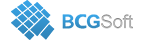BCGControlBar Pro for MFC
 CBCGPChartObject::CBCGPChartObject ( CBCGPChartVisualObject * pParentChart, const CBCGPRect & rcCoordinates, CoordinateMode mode = `CM_PIXELS` )

Constructs a CBCGPChartObject object.

The constructor. mode can be one of the following enumerated values:

• CM_PERCENTS - members of rcCoordinates are treated as offsets from left, right, top and bottom bounds of the Chart calculated in percents from the Chart size.
• CM_PIXELS - members of rcCoordinates are treated as offsets in pixels from the Chart bounds.
• CM_CHART_VALUES - members of rcCoordinates are treated as values on related X (left, right) and Y (top, bottom) axes.
• CM_CHART_VALUE_DIST_ANGLE - rcCoordinates.left - X value, rcCoordinates.top - Y value, rcCoordinates.right - distance, rcCoordinates.bottom - angle.
• CM_AXIS_INSIDE_MARK - rcCoordinates.left - X value, rcCoordinates.top - Y value, rcCoordinates.right - distance in pixels from the point defined by X or Y axis values, rcCoordinates.bottom - distance in pixels from the related axis.
• CM_AXIS_OUTSIDE_MARK - the same as CM_AXIS_INSIDE_MARK, but the distance is calculated in opposite direction.
Parameters
 pParentChart A pointer to a parent chart visual object. rcCoordinates Object coordinates. mode Coordinate mode.